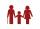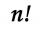# Quadratic equation + functions - math problems

#### Number of problems found: 92

• Young mathematicianOne young mathematician was bored again. He found that the average age of people in the room where the seminar equals to its count. Then his 29-year-old brother entered this room. Even then, the average age of all present was the same as the count of peop
• Find twoFind two consecutive natural numbers whose product is 1 larger than their sum. Searched numbers expressed by a fraction whose numerator is the difference between these numbers and the denominator is their sum.
• Digit sumThe digit sum of the two-digit number is nine. When we turn figures and multiply by the original two-digit number, we get the number 2430. What is the original two-digit number?
• Two workersTwo workers should fulfill certain task together for 5 days. If the first worker increased their performance twice and second twice fell, it took them just four days. For how many days would handle the entire task first worker himself?
• Wagons and cranesSeveral of the same cranes unloaded 96 wagons. If there were 2 more cranes there would be less 8 wagons for each crane. How many cranes were here?
• Average ageThe average age of all people at the celebration was equal to the number of people present. After the departure of one person who was 29 years old, average age was again equal to the number present. How many people were originally to celebrate?
• VariableFind variable P: PP plus P x P plus P = 160
• VCP equationSolve the following equation with variations, combinations and permutations: 4 V(2,x)-3 C(2,x+ 1) - x P(2) = 0
• ElementsIf the number of elements is decreased by two the number of permutations is decreased 30 times. How many elements are?
• CombinationsIf the number of elements increase by 3, it increases the number of combinations of the second class of these elements 5 times. How many are the elements?
• Rectangle poolFind dimensions of an open pool with a square bottom with a capacity of 32 m3 to have painted/bricked walls with the least amount of material.
• Cuboid - ratiosThe sizes of the edges of the cuboid are in the ratio 2: 3: 5. The smallest wall has an area of 54 cm2. Calculate the surface area and volume of this cuboid.
• Rectangle diagonalsIt is given a rectangle with an area of 24 cm2 a circumference of 20 cm. The length of one side is 2 cm larger than the length of the second side. Calculate the length of the diagonal. Length and width are yet expressed in natural numbers.
• Paper boxThe hard rectangular paper has dimensions of 60 cm and 28 cm. The corners are cut off equal squares, and the residue was bent to form an open box. How long must beside the squares be the largest volume of the box?
• Four integersFnd four consecutive integers so that the product of the first two is 70 times smaller than the product of the next two.
• Difference of two numberThe difference between the two numbers is 20. They are positive integers greater than zero. The first number raised to one-half equals the second number. Determine the two numbers.
• TrapeziumThe length of the base and the height size of the base of the trapezium is at ratio 5:3:2, the content area of the trapezium is 128 cm2. Calculate the length of the base and the height of the trapezoid.
• SquareSuppose the square's sides' length decreases by a 25% decrease in the content area of 28 cm2. Determine the side length of the original square.Which of the points belong function f:y= 2x2- 3x + 1 : A(-2, 15) B (3,10) C (1,4)Write the equation of the quadratic function, which includes points A (-1, 10), B (2, 19), C (1,4)